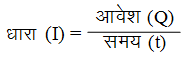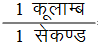Ex﻿

# 1. Electricity

#### Electric Current (Charge In Motion)

Definition : The quantity of electric charge flowing through cross section of a given conductor in one second is called current.
Thus, if Q is the charge which flows through a conductor in time t, then the current (I) is given byThe electric current (or current) is a scalar quantity.
Unit of current
The SI unit of charge (Q) is coulomb (C), and that of time (t) is second (s). So,
SI unit of current= 1 Ampere
The unit coulomb per second (Cs–1) is called ampere (A)
Direction of Electric Current :
The direction of flow of the positive charge taken as conventional direction of the electric current.
When we consider the flow of electric current in an ordinary conductor, such as a copper wire, the direction of current is taken as opposite to the direction of the flow of electrons.

If you want to give information about online courses to other students, then share it with more and more on Facebook, Twitter, Google Plus. The more the shares will be, the more students will benefit. The share buttons are given below for your convenience.
×

#### NTSE Physics (Class X)

• Electricity
• Magnetic Effect of Curent
• Light Reflection
• Light Refraction
• Human Eye & Colourful World
• Source of Energy
• Motion

#### NTSE Physics (Class IX)

• Force & laws of motion
• Work and Energy
• Gravitation
• Sound

SHOW CHAPTERS